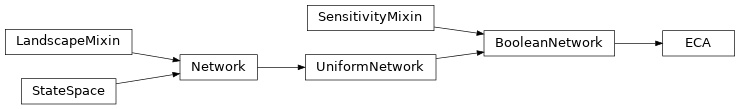# Elementary Cellular Automata¶

The neet.boolean.ECA class describes an Elementary Cellular Automaton with an arbitrary rule.

class neet.boolean.ECA(code, size, boundary=None, names=None, metadata=None)[source]

ECA represents an elementary cellular automaton rule. Each ECA contains an 8-bit integral member variable code representing the Wolfram code for the ECA rule and a set of boundary conditions which is either None, signifying periodic boundary conditions, or a pair of cell states signifying fixed, open boundary conditions. As with all neet.Network classes, the names of the nodes and network-wide metadata can be provided.In addition to all inherited methods, ECA exposes the following properites:

 code The Wolfram code of the elementary cellular automaton. boundary The boundary conditions of the elemenary cellular automaton.
Parameters: code (int) – the Wolfram code for the ECA size (int) – the size of the ECA’s lattice boundary (tuple or None) – the boundary conditions for the CA names (seq) – an iterable object of the names of the nodes in the network metadata (dict) – metadata dictionary for the network ValueError – if code is not in $$\{0,1,\ldots,255\}$$ ValueError – if boundary is a neither None nor a pair of binary states
code

The Wolfram code of the elementary cellular automaton.

Examples

>>> eca = ECA(30, size=5)
>>> eca.code
30
>>> eca.code = 45
>>> eca.code
45
>>> eca.code = 256
Traceback (most recent call last):
...
ValueError: invalid ECA code

Type: int ValueError – if code is not in $$\{0,1,\ldots,255\}$$
boundary

The boundary conditions of the elemenary cellular automaton.

Examples

>>> eca = ECA(30, size=5)
>>> eca.boundary
>>> eca.boundary = (0, 1)
>>> eca.boundary
(0, 1)
>>> eca.boundary = None
>>> eca.boundary
>>> eca.boundary = [0, 1]
Traceback (most recent call last):
...
TypeError: ECA boundary are neither None nor a tuple

Type: tuple, None ValueError – if boundary is a neither None nor a pair of binary states